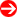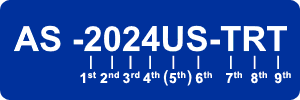#Product Naming Convention - Servers (AMD)Character Representation Options 1st Form Factor • 1 = 1U         • 2 = 2U         • 3 = 3U         • 4 = 4U / Tower 2nd HD Tray Type / Chassis • 0 = 3.5"         • 1 = 2.5" 3rd CPU Count • 1 = Single         • 2 = Dual         • 4 = Quad 4th Generation • 3 = 4th Gen. (Socket SP3; Zen)         • 4 = 5th Gen. (Socket SP3; Zen 2) (5th) Server Platform • BT = BigTwin™         • G = GPU         • TP = TwinPro™         • U = Ultra Server 6th MB Platform / Chipset • S = Socket SP3 Board (Zen / Zen 2) 7th/8th Interface Type ------------------------------- All Generations ------------------------------         • C0 = SAS 12G/s (HBA)         • C1 = SAS 12G/s (HW RAID)         • H(B) = (Twin hot-plug)         • H(D) = Dual node twin hot-plug         • L = Cost-Effective         • M = Short depth < 20" chassis         • N = NVMe         • N10 = 10 NVMe         • N24 = 24 NVMe         • T = SATA         • TR4 = 4x 1G         • TR25B = 25G         • TRT = 2x 10G 9th and higher Power Redundancy / IPMI • R = Redundant Power